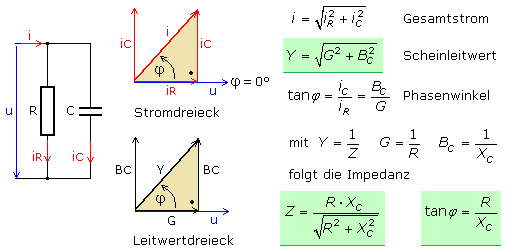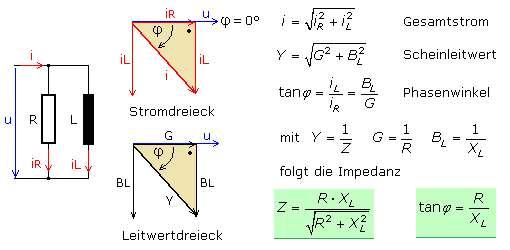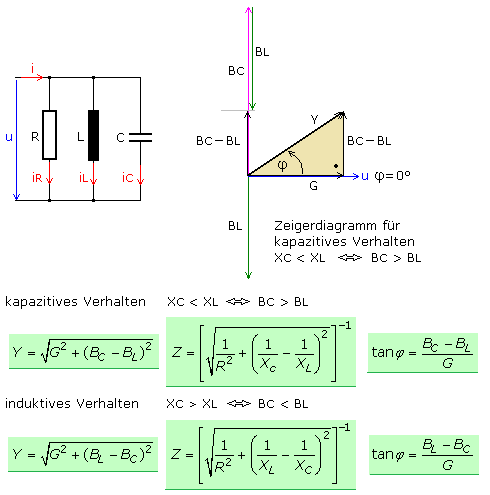# What is Z in an RLC circuit

## RLC vector diagrams in the AC circuit

### RC parallel connection

In a parallel circuit, resistors are all connected to the same voltage, which becomes the reference value in the vector diagram. The voltage vector points in the x-axis direction with the angle φ = 0 °. The current indicator at the effective resistance points in the same direction, since the phase angle is 0 °. The current leads by + 90 ° at the capacitive reactance and the current vector points in the positive y-axis direction.

A right-angled current triangle can be drawn. The total flow is calculated from the geometric addition of the partial flows. The phase angle between voltage and current results from tanφ, the quotient of reactive component to active component. The partial currents are directly proportional to the conductance values. The current triangle can consequently be redrawn into a proportional conductance triangle. The conductance values ​​can be replaced by the reciprocal values ​​of the corresponding resistance values.### RL parallel connection

The AC voltage applied to the parallel connection is the reference value. At the effective resistance, voltage and current are in phase with φ = 0 °. At the inductive reactance of the coil, the current lags the voltage by φ = −90 °. The mathematical relationships can easily be derived from the vector diagram.### RCL parallel connection

The reference variable with φ = 0 ° is the alternating voltage. The voltage and current at the ohmic resistance are in phase. At the capacitive reactance the current leads by φ = + 90 ° and at the inductive reactance by φ = −90 °. The current triangle and, derived from it, the conductance triangle can be drawn by corresponding parallel displacement of the pointers.The phase angle can assume positive or negative values, depending on whether the inductance or the capacitance and their values ​​determine the character of the circuit. If both reactive components are of the same size, the RCL parallel connection acts like an ohmic resistor with φ = 0 ° to the outside. This case is described in detail in the chapter on parallel resonant circuit.